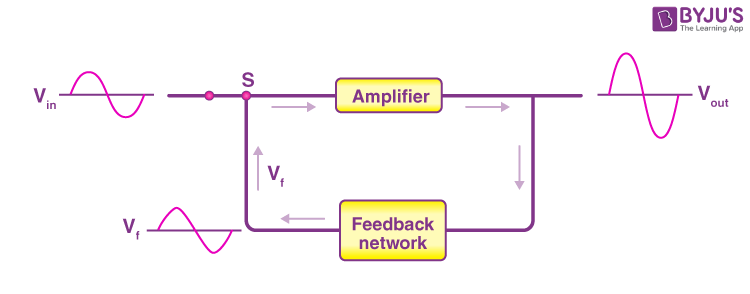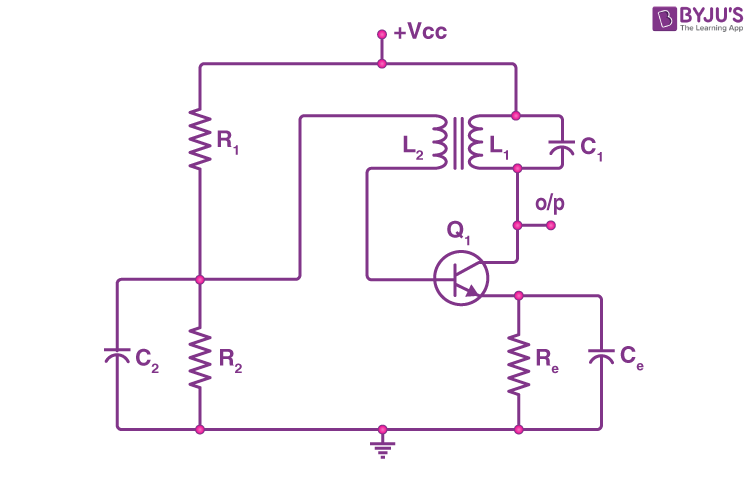# Feedback Amplifier and Transistor Oscillator

In an amplifier, we have seen that a sinusoidal input is given which appears as an amplified signal in the output. This means that external input is necessary to sustain an AC signal in the output for an amplifier. In an oscillator, we get AC output without any external input signal. In other words, the output in an oscillator is self-sustained. To attain this, an amplifier is taken.Consider the circuit in the image shown above. Here we observe that apart from the input signal, a portion of the output signal is sent back to the input line. Here the potion is termed as the feedback to the system is sent such that it is either in-phase (positive feedback) or out-of-phase (negative feedback) with the starting input signal.

## Working of an Oscillator

Let us now understand the working of an oscillator with the help of a circuit, as shown below.In this circuit, the feedback network includes an inductor and a capacitor, and the feedback is accomplished by inductive coupling from one coil winding to another coil. Here the coils are inductively coupled through mutual inductance as the coils are wound to the same core. Here, the base-emitter junction is forward biased and the base-collector junction is reverse biased.

Now, when the switch S1 is put on to apply a proper bias for the first time, collector current flows in the transistor through the second inductor given by L2. This current initiative is not at its full amplitude but increases to the highest value with time. The electric current now flows through in the emitter circuit due to the inductive coupling between the coils L1 and L2, which is actually the feedback from the input to the output in this circuit. The feedback as we see here is positive and hence the current increases in the emitter circuit. When the transistor becomes saturated, the current in the collector circuit increases to its maximum value after which it can increase no further. Since the current cannot increase any further, the increase in the magnetic field around L2 ceases. And as the field becomes static, the feedback from the inductor L2 to the inductor L1 stops. Thus, as the feedback stops, the emitter current begins to fall, and thus the collector current decreases, due to which the magnetic field around the coil L2 starts to decay and further because of this, the inductor L1 witnesses a decaying field in L2. As a result of this, when the power is cut off, the emitter current decreases to a value Z’. The transistor is said to have reverted from its original state. The same process repeats itself again and again. The frequency with which the oscillator goes from the state of saturation to the state of cut-off and back to the saturation state is termed as the resonance frequency and is given as,

$$\begin{array}{l} \nu = \frac{ 1 }{ 2 \pi \sqrt{ L C } } \end{array}$$

The circuit under consideration is termed as a tuned collector oscillator as the tuned circuit has been connected to the collector side the tank or tuned circuit is connected to the collector side.

## Frequently Asked Questions – FAQs

Q1

### What are semiconductors?

Semiconductors are the materials which have a conductivity between conductors (generally metals) and non-conductors or insulators (such as ceramics). Semiconductors can be compounds such as gallium arsenide or pure elements, such as germanium or silicon.
Q2

### What is a transistor?

A transistor is a type of a semiconductor device that can be used to both conduct and insulate electric current or voltage. A transistor basically acts as a switch and an amplifier.
Q3

### Name the three basic terminals of a transistor.

There are three terminals for a transistor. They are:
• Base: This is used to activate the transistor.
• Collector: It is the positive lead of the transistor.
• Emitter: It is the negative lead of the transistor.
Q4

### What is valence band?

The energy band involving the energy levels of valence electrons is known as the valence band. It is the highest occupied energy band. When compared with insulators, the bandgap in semiconductors is smaller.
Q5

### How does a transistor work as an amplifier?

A transistor works as an amplifier by taking in a very small weak signal through the base junction and raising the strength of the weak signal. This amplified signal is released through the collector.

Stay tuned to BYJU’S and Fall in Love with Learning!

Test Your Knowledge On Feedback Amplifier Transistor Oscillator!